## Tuesday, July 17, 2012

### Solving the Fourth Degree: Part II

When we left off, we had just reached the halfway point in our solution of the quartic equation. What we had done was to show the steps necessary to write explicit expressions p, q, and r for these three peculiar functions of alpha, beta, gamma and delta: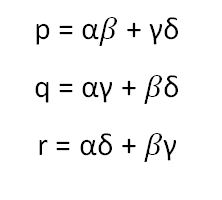The p, q and r turned out to be the roots of a cubic with rational coefficients that we can easily calculate. Our alphas and betas etc. are of course the roots of our quartic. Now let's see how we can extract them individually from our p's and q's etc.

The next step is to consider all possible combinations of the alphas and betas etc. with alternating sign: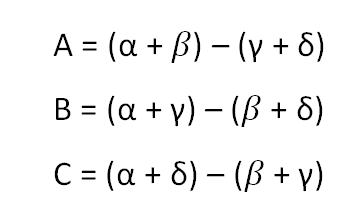There are just three more possible combinations, but they amount to what we already have with opposite signs. What I want to show you now is that our A, B, and C are actually the square root of numbers that we have access to via our p's and q's etc! You can verify this by squaring A for example: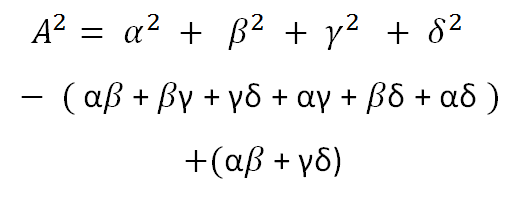Look what we have. The first line in alpha-squared etc. is symmetric in alpha-beta-gamma-delta, so it's just a rational number. The second line in alpha-beta etc. is also symmetric...in fact, it's just the coefficient of the linear term in the quartic equation. So it's also a rational number. The third line is our p, the number we said was the solution of a cubic equation for which we know the coefficients. So in other words, our A is just the square root of (p + b), where b is a rational number.

It's obvious that our B and C are similarly just the square roots of similar functions which we can write in radicals. So we can write A, B, and C explicitly...at least up to the ambiguity of a minus sign. But look what we get for the sum of A, B, and C: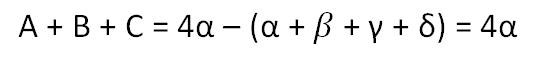By adding up A, B, and C we isolate alpha! Remember it's easy to make the second-from-leading coefficient in any polynomial equal to zero by a simple linear shift, which knocks out the bracketed term in the above expression. (It's called "completing the square" when we do it on a quadratic equation.) We've shown an explicit expression which solves the quartic equation.

What about beta, gamma and delta? Remember there was an ambiguity when we took square roots. We might have chosen alternate signs in each case. The different choices for the sign of the square root lead to sums of A, B and C which are variously beta, gamma and delta depending on our choices.

And that's how you solve the fourth-degree equation.

### Solving the Fourth Degree: Part 1

Today I'm going to try and write about the picturesque and mysterious way that the fourth degree equation is solvable. I've been using the Greek letters to stand for the roots of an equation, so the roots of the fourth degree will be alpha, beta, gamma, and delta. The factored equation can therefore be written explicitly as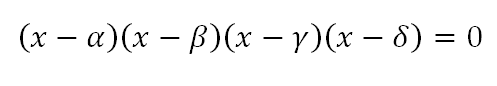When you multiply this out, you get the coefficients of the polynomial as the elementary symmetric functions in alpha, beta, gamma, and delta: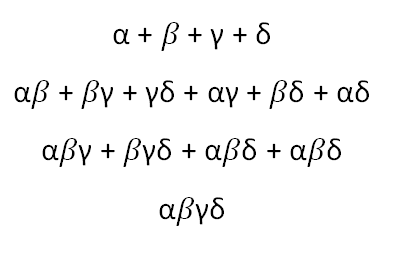What makes the fourth degree equation solvable is the existence of this unique trio of functions in alpha, beta, gamma and delta:If we consider the splitting field of the polynomial, which is what I'm going to call the algebraic completion of the rationals formed by adjoining the four roots and taking all resulting algebraic combinations of those elements, it is clear that our p, q, and r reside within the splitting field. What is interesting is that they themselves are the roots of a cubic equation with rational coefficients! We can easily see why this must be so.

In the most general case, we cannot distinguish algebraically between the four roots of our quartic equation. If I state a true fact about alpha and beta, then you can turn around and state the same fact about gamma and delta, and as long as we restrict ourselves to algebraic manipulations whose final result is a rational number, then your fact will be just as true as my fact. In other words, we can freely shuffle our alpha, beta, gamma and delta and every algebraic statement is just as true before and after the shuffling.

Now, is we shuffle our four roots and consider the impact on our p's, q's, and r's, it is easy to verify the interesting result that any shuffling whatsoever will do one of two things: it will leave our p's and q's etc. untouched....or, it will swap them about with each other. In the even that there is swapping, it may be that just two of them get swapped, or it may be that all three are rotated with each other.

Furthermore, the property of indistinguishability with respect to shuffling of the four roots of the quartic is inherited by our p's and q's etc: a true statement about p, q, and r remains true if we arbitrarily shuffle them around. But this is just the property which characterises the roots of a cubic equation.

In fact, we can see explicitly that our p's and q's etc. are indeed the solution of a cubic equation by considering their elementary symmetric functions:

p + q + r

pq + qr + rp

pqr

These three functions are just the coefficients of the cubic equation of which p, q and r are the roots. We just need to substitute their definitions in terms of the alphas and betas etc. to verify that they are indeed rational numbers. This follows from the easily verified observation that they are symmetric in alpha, beta etc.

We've already seen that a cubic solution is solvable in radicals, and we talked about how to write the solution, That means we can get explicit expressions for p, q, and r.

We are now halfway to solving the quartic equation.